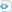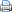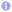Virtual Functions Considered Not Harmful, Part II

For the lack of a profiler (and for the lack of time), I commited to the cardinal sin of making unproven statements in Virtual Functions Considered Not Harmful, Part I

 Original post by dascandy call *%eax call __ZNK3Bar4fun2Eii The first causes (often) a pipeline stall. The second never does. Please try to *profile* your speed tests before making any statements. You’re not working on 386′s, 486′s or Pentiums, your CPU is not trivial anymore.

The key concepts here are the Branch History Table (as in “your CPU is not trivial anymore” [sic!]) on modern CPU and the fact that the advantage of non-virtual functions decreases with increasing complexity of the function itself.

You are right, I should have used a profiler, but unfortunately, I don’t have one handy on this box. Hence I wrote the following benchmark (mostly Std-C++ compatible, except for the asm()’s  and the __attribute(())’s for the sake of benchmarking).

Benchmark

I tried to be as careful as possible, if I have done something bogus, please let me know. So, here it is:

```Code block#ifdef __GNUC__
#define MARK(x) do{asm volatile ("# "#x);}while(0)
#define NO_OPTIMIZE_AWAY() do{asm("");}while(0)
#else
#error "dies"
#endif

#include <ctime>
#include <iostream>
#include <cmath>

struct StaticNoInline {
float dist (float const volatile *lhs, float const volatile *rhs)
__attribute__((noinline)) {
float const diff [] = {
rhs  - lhs ,
rhs  - lhs ,
rhs  - lhs ,
};
float const lenSq = diff *diff  + diff *diff 
+ diff *diff ;
return sqrt (lenSq);
}
};

struct StaticForceInline {
float dist (float const volatile *lhs, float const volatile *rhs)
__attribute__((forceinline)) {
float const diff [] = {
rhs  - lhs ,
rhs  - lhs ,
rhs  - lhs ,
};
float const lenSq = diff *diff  + diff *diff 
+ diff *diff ;
return sqrt (lenSq);
}
};

struct IVirtual {
virtual float dist (float const volatile *lhs, float const volatile *rhs)
__attribute__((noinline)) = 0;
};

struct Virtual : public IVirtual {
float dist (float const volatile *lhs, float const volatile *rhs)
__attribute__((noinline)) {
float const diff [] = {
rhs  - lhs ,
rhs  - lhs ,
rhs  - lhs ,
};
float const lenSq = diff *diff  + diff *diff 
+ diff *diff ;
return sqrt (lenSq);
}
};

template <typename T, typename I, uint32_t count>
void test () {
static volatile float dist;
static volatile float lhs ;
static volatile float rhs ;

::std::cout << "Beginning test for " << typeid (T).name()
<< ", count is " << count
<< 'n';

I &subject = *new T ();
clock_t const beginT = clock();
MARK("entering loop");
for (uint32_t i=0; i<count; ++i) {
dist = subject.dist (lhs, rhs);
}
MARK("left loop");
clock_t const endT = clock();
delete &subject;
::std::cout << "Test ended, total time " << endT - beginT << "msec.n";
}

int main () {
const uint32_t count = 1<<27;
test<Virtual, IVirtual, count> ();
test<StaticNoInline, StaticNoInline, count> ();
test<StaticForceInline, StaticForceInline, count> ();
// Re-execute to take care of CPU heat and priority switches
test<Virtual, IVirtual, count> ();
test<StaticNoInline, StaticNoInline, count> ();
test<StaticForceInline, StaticForceInline, count> ();
::std::cout << ::std::flush;
}```

Basically, this benchmark calculates many million vector3-lengths.

Results

On a Pentium(R) M, 1.8GHz, the program gives me really debunking numbers.

stdout of benchmark

```  Beginning test for 7Virtual, count is 134217728
Test ended, total time 5828msec.
Beginning test for 14StaticNoInline, count is 134217728
Test ended, total time 5839msec.
Beginning test for 17StaticForceInline, count is 134217728
Test ended, total time 5118msec.
Beginning test for 7Virtual, count is 134217728
Test ended, total time 5798msec.
Beginning test for 14StaticNoInline, count is 134217728
Test ended, total time 5809msec.
Beginning test for 17StaticForceInline, count is 134217728
Test ended, total time 5097msec.```

interpretation

clock() is not too exact (especially on Windows(R)), so I ran it for over 5 seconds (134,217,728 loops). In more readable form, the results are:

• virtual: 5.828 sec, 5.798 sec = 5.813 sec on average
• static, not inlined: 5.839 sec, 5.809 sec = 5.824 sec on average
• static, inlined: 5.118 sec, 5.097 sec = 5.1075 sec on average

The static and the virtual variant run at nearly the same speed (roughly 5.8185 sec on average), the inlined version runs in only roughly 87.78 % of the time.

Upshot

Certainly, inlining helps with a bunch of other optimizations, like auto-vectorisation or re-use of data, plus it increases locality, but has the disadvantage of potential code-bloat.

Also, at the time where the compiler decides to not inline a function, the performance difference between virtual and non-virtual decreases (w.r.t. several parameters and configurations), especially in tight loops. So if it would be a great benefit for your application to use virtual functions, then you might decide to use them.

Example for a “great benefit” of virtual functions

Just to give an example (blatant self promotion, sorry), my ray tracer picogen uses virtual functions for one of its shader systems (basically an executable abstract syntax tree; the performance drop is not too big as the shader is only called for definitive correct intersections, where in the meanwhile many other operations happened), plus it uses virtual functions for its intersectable objects (which include a quadtree, a BIH, oops that was the ray tracer I wrote before that, sorry, spheres of course, a cube, a simple DDA based heightmap, and some hacks where I tried things out). The general BIH is an aggregate that can house any other type of Intersectable, including specific and general BIH. Also, I implemented a Whitted-style Ray Tracer and a Path Tracer (called “Surface Integrators”, like pbrt calls it), which definitely are called for each pixel, even if there is no intersection at all.

Without virtual functions, this all would have been far clumsier, and not necessarily faster. Had I organized those objects in multiple lists, code could have even run slower than with virtual functions.

This entry was posted in C++ and tagged , , , , . Bookmark the permalink.